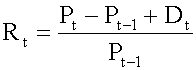Tags
Question
Holding period return formulaTags
Question
Holding period return formula

?

Tags
Question
Holding period return formulaIf you want to change selection, open document below and click on "Move attachment"

Subject 2. Holding Period Return
When analyzing rates of return, our starting point is the total return, or holding period return (HPR). HPR measures the total return for holding an investment over a certain period of time, and can be calculated using the following formula: P t = price per share at the end of time period t P (t-1) = price per share at the end of time period t-1, the time period immediately

#### Summary

status measured difficulty not learned 37% [default] 0

No repetitions